Thermodynamics and Propulsion

# 13.4 Aircraft Endurance

If the time spent in the air is of interest and not the distance traveled then one is concerned with endurance. The maximum endurance of an aircraft (or the time aloft) refers to a flight condition that requires the minimum fuel power. Assuming that the overall propulsion system efficiency,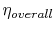, defined as the propulsive power over the fuel power, is constant, maximum endurance can be obtained by minimizing the required propulsive power or rate of energy expenditure,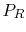, as shown in Figure 13.3.

We can determine the aerodynamic configuration which provides the minimum energy expenditure: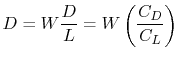sowhereThenSo the minimum power required (maximum endurance) occurs when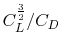is a maximum. With a little algebra we can arrive at an expression for the maximum endurance. Setting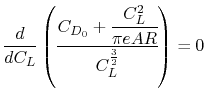we find thatand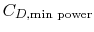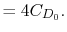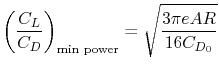and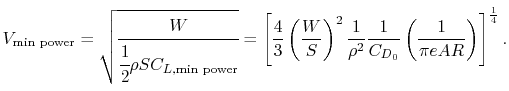Thus the minimum power (maximum endurance) condition occurs at a speed which is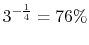of the minimum drag (maximum range) condition. The corresponding lift-to-drag ratio is 86.6% of the maximum lift-to-drag ratio, shown in Figure 13.5.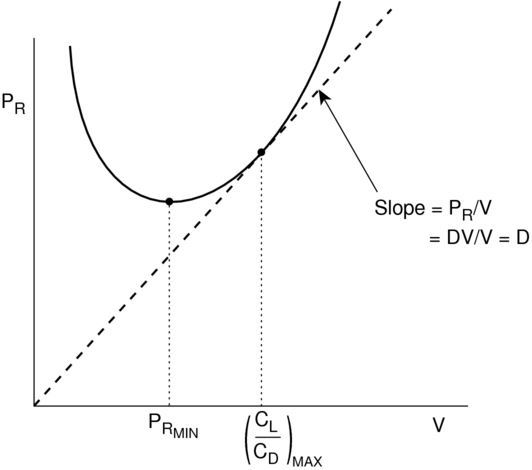Continuing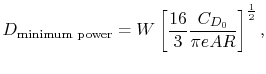which can be substituted into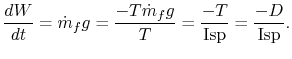Such that, for maximum endurance,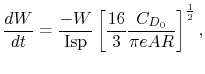which can be integrated (assuming constant Isp) to yieldUnifiedTP